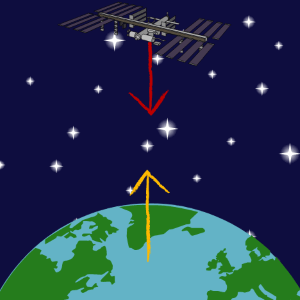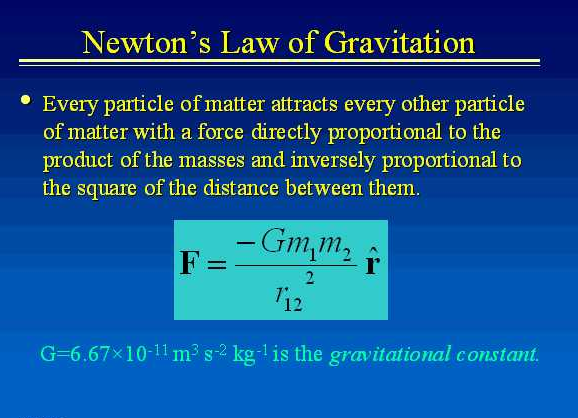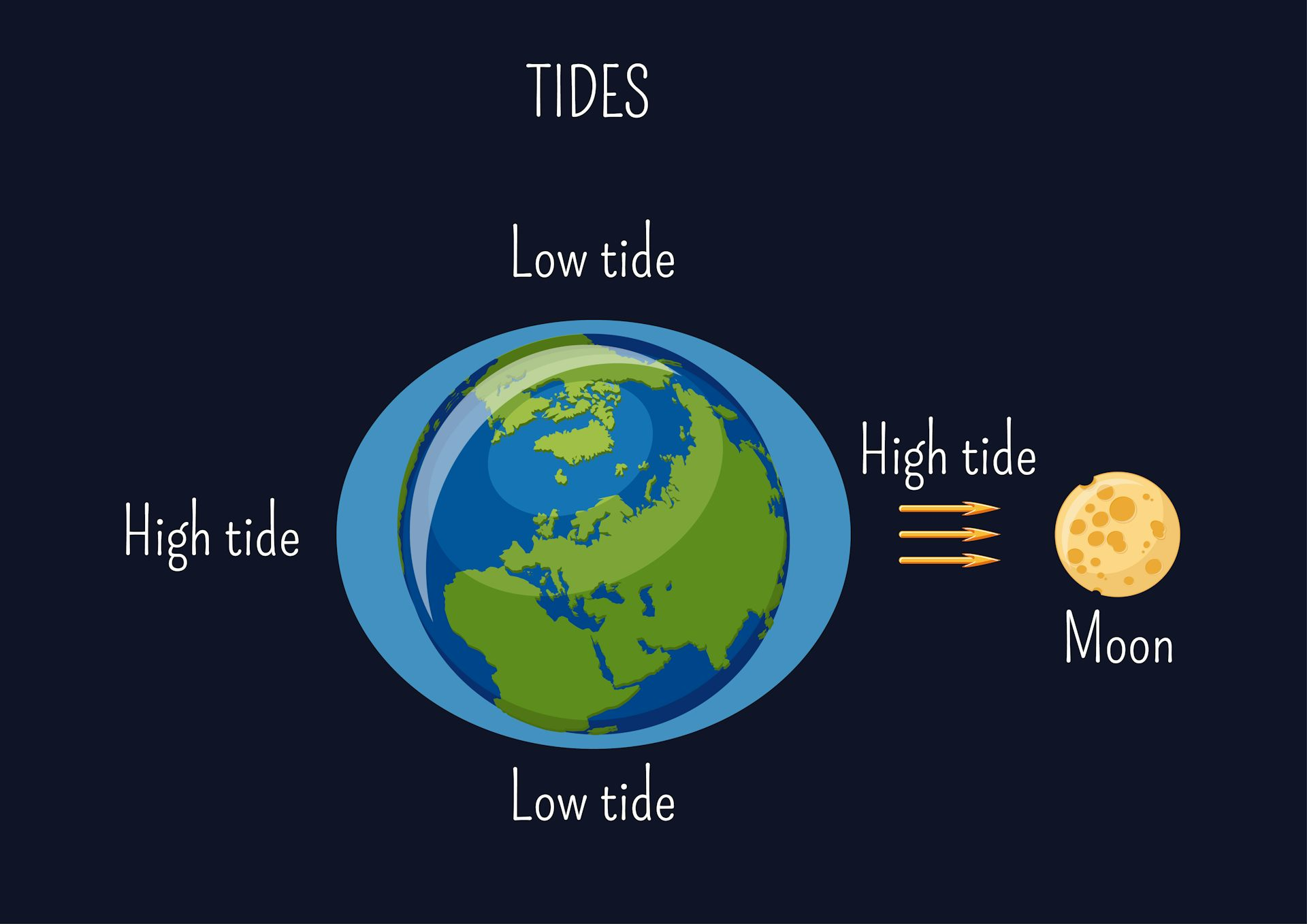# Gravitational Force Calculator- Formula, Definition, Examples and, the complete guide

Everybody knows the story of an apple falling off and hitting Sir Isaac Newton’s head. This phenomenon is what led to the discovery of gravity.

Somehow, that esteemed apple tree remains in Newton’s home garden at his birthplace in Lincolnshire. Sir Isaac Newton and the apple tree, both have equal shares in their contribution to gravity.### Gravitational ForceThere is a force of attraction that exists between any two objects on every planet in the solar system. Most importantly, each time we jump, a force pulls us down and that is the gravitational force at work.

However, if you want to experiment, here’s a fun one. Throw a piece of cake high up in the air, and you will see it come crashing on the ground. That is going to hurt you and never let you forget the existence of the ‘gravitational force’.

Now, hold on to this thought for a moment. We are going to use this for another later discussion.

### Gravitational force Definition

Gravitational force is the force of attraction between all masses in the universe; especially the attraction of the earth’s mass for bodies near its surface. It is one of the four fundamental forces of physics. These forces provide the basis for everything else. The other three fundamental forces are the strong force, the weak force and, the electromagnetic force.

### Newton’s Law of Universal Gravitation

Do you remember the experiment with cakes that we suggested earlier?

Drawing from that above discussion, we can broaden its base by saying that the same happens for every object on earth. Subsequently, it is this gravitational force that stops the sun, moon and, other heavenly bodies from falling on one another.

So we can conclude that studying this force is of utmost importance.

Newton’s law of Universal Gravitation suggests nothing different. It states that every object, including you, exerts a force of pull on every other object of the universe. Thus, it simply shows the universality of gravity. Newton’s discovery that gravity is universal, earned him his place in the Gravity Hall of Fame.

### Gravitational Force formula

So far, we have got to know what gravity is and how it acts on every object in the universe. Now, the curious minds out there would like to know its quantitative aspect. That is, what is the measure of the gravitational force that is working between any two bodies placed near one another?

To answer this question, we need to make some calculations. Hence, we introduce Newton’s law of Gravitation.

#### Newton’s law of Gravitation

On comparing the acceleration of the moon to the acceleration of objects on earth, Newton some facts. He believed that this gravitational force depended greatly on the distance between objects. He concluded that the gravitational force acting between any two objects is inversely proportional to the distance between the centers of the said objects.

He also realized that this force was not dependent only on ‘distance’.

For example, when a ball is thrown upwards, a gravitational force acts on it. This force varies inversely with the distance between the center of the ball and the center of the Earth. It is also dependent on the mass of the ball which leads us to Newton’s famous equation on ‘force’:

F (net) =m*a,

Where ‘m’ is the mass of the object and ‘a’ is its acceleration.

According to Newton’s third law, when two bodies interact, they apply forces on one another. These forces are equal in magnitude and opposite in direction.

Consequently, the force acting to cause the apple’s downward acceleration also causes the earth’s upward acceleration. Thus, it becomes easy for us to conclude that the gravitational force acting between the earth and the ball also depends on the mass of the earth.

Thus, there is a direct dependence between the gravitational force acting between bodies and the product of their masses.

Its vector representation will be as follows:#### Gravitational Force Equation

This gravitational force of attraction is directly dependent upon the masses of both objects and inversely proportional to the square of the distance that separates their centers. Newton’s conclusion about the magnitude of gravitational forces is summarized as:Where,

‘F1’= ‘F2’= F (gravitational force),

‘m1’ is the mass of the first object,

‘m2’ is the mass of the second object,

The two objects are separated by the distance ‘r’ and,

‘G’ is the constant of proportionality or the GRAVITATIONAL CONSTANT (which we have discussed later)

Thus, from this equation, we can clearly see that for calculating the gravitational force between two objects, we just need to know their respective masses and the distance of separation of their centers. The calculation is pretty simple actually.

From the formula, we can say that for bodies having huge masses, the gravitational force acting between them will also be massive. Conversely, for bodies with smaller masses, the force of attraction acting between them will also be smaller.

Similarly, if two bodies are placed far apart, the gravitational force of attraction between them will be minimal and vice-versa.

The doubling of mass of any one of the two bodies doubles the force acting between them, whereas,

The gravitational force of attraction between two bodies will decrease by a factor of 4 if the distance between two bodies is doubled.

#### Gravitational Force Constant (G)

Much like all other constants used in Physics, the gravitational constant is an empirical value. It was found by Henry Cavendish via experiments in the century after Newton’s death.

#### Gravitational Constant value:

The image below shows the empirical value of G:Though the unit in which G is expressed looks a bit complex, it can be simplified. If we place it in the above equation, then the final result is expressed in terms of newton (N).

Its value does not change in any part of the universe and it doesn’t depend on the masses of the bodies at all. Hence, it is called a universal constant.

### Gravitational force calculator

#### What is gravitational force calculator?

The Gravitational Force calculator is a simple online tool that anyone can use to derive the value of the gravitational forces acting between any two bodies.

It follows quite a convenient process.

We already know the formula required to calculate the gravitational force of attraction between bodies.

However, if we use the gravitational Force calculator, we need not memorize any formula at all. We must know the respective masses of the two bodies and the distance by which they are separated.

The calculator provides spaces. You just have to pop in these values and there, the result is displayed. It can be expressed in whichever unit of force you prefer. The available options of the units of Force in which you can obtain the results are:

Newtons (N), kilo newtons (kN), mega newtons (mN), giga newtons (gN), tera newtons (tN), pounds-force (lbf).

## Gravitational Force Calculator with steps

Step-1: Enter the masses of the two bodies (m1 and m2) in the space provided.

Step-2: Enter the distance(r) between the two bodies.

Step-3: Click on the ‘submit’ button to calculate the gravitational force.

Step-4: The gravitational force is shown in the output box.

You can choose any from the wide range of options available on the internet. You can also simply click on the link below to calculate the gravitational force between bodies:

Gravitational Force Calculator

### Why use Gravitational Force calculator?

Anyone who wishes to calculate the gravitational force acting between any two bodies can use the gravitational force calculator.

• The gravitational force calculator is very easily available on the internet.
• The process is quite simple and hassle free.
• Gives you accurate results every single time.
• Saves time, in comparison to the pen-and-paper-based calculation method.

To summarize, the gravitational force calculator is an amazing tool that can make gravity calculations a lot easier for you.

#### Gravitational force examples

Whenever we look around us, we see the force of gravity in play. Every object with non-zero mass exerts a pull on any other object having non-zero mass.

1. A force of gravity is acting between you and the computer screen at the moment while you are reading this. This force of attraction is quite small and hence we are not able to sense it.
2. On the contrary, this is not what happens in the case of large heavenly bodies. When we shift our focus to heavenly bodies like the sun, the moon, or the planets, things change. The effects of gravitational force begin to show clearly. This is because these bodies have a huge mass. And since the force is directly proportional to the product of the masses of the bodies gravitational force increases.
3. In the case of a plane crash, do you know who the real villain would be?

Neither the pilot nor the captain, but GRAVITY. If there was no gravitational force at play, then the plane would probably float mid-air. Thus, it would save  the lives of many.

### Gravitational Force in Nature1. It is due to gravity that tides occur. There are two types of tides: low tides and high tides. Tides are caused by the gravitational forces exerted on the earth by the moon. Sometimes, even due to the sun. The part of the ocean the moon faces, bulges out. It is because the gravitational pull of the moon is strongest on whichever side of the Earth faces it. Its opposite side, which does not directly face the moon, has a stronger effect of inertia and hence it also bulges outwards. Therefore, this leads to the occurrence of high tides on these two sides. At the same time, the two other sides of the earth experience low tides since they do not bulge outwards.1. For those of you who enjoy star-gazing, did you know that their twinkling lights are a consequence of gravity?

The formation of stars is a pretty complex process.

Initially, in outer space the swirling clouds of cool gas collapse under the huge force of gravity. Thus making particles collide at a faster rate, ultimately generating a lot of heat. The gravitational forces acting at the center of the swirl are very strong. And thus, they cause the atoms to move fast enough and fuse. When the nuclei of these atoms smash together, it releases a huge amount of energy. This, in turn, makes the stars shine brightly.

#### Gravitation hypothetical applications

• Are you losing your sleep over weight-gain issues? Or, can you not eat a pizza in peace just because you keep counting your Calories?

Well, stop worrying about something so superficial. The moment you leave earth and step on Mars, you will have your weight slashed to a mere 38% of what it was earlier. This would allow you to binge-watch another episode of your favorite TV series with a bowl of chicken nuggets.

According to Elon Musk,a Martian Society will soon be in-the-making. Let us hope for that to happen soon enough!

• For as long as we are on the surface of the earth, we cannot escape the tantrums of gravity. So if you want to get rid of the gravitational force once and for all, there is a way. You could surely ask someone to launch you from the earth’s surface with a velocity greater than roughly 11.2km/s.

That my friend is the escape velocity of the earth. Launching you with this velocity could help you escape from the gravitational center of attraction of the earth. That too without undergoing any further acceleration.In conclusion, much like anything else, Gravitational force is not all good or all bad. It is a pivotal force that has kept life as we know it, in existence. It has established connection among every person and every object on Earth.

#### Frequently asked questions on gravitational force

##### What is Earth’s gravitational force?

The Gravitational force of earth is 9.807 m/s2.

##### Gravitational force of earth is maximum at?

Gravitational force of earth is maximum at the poles.